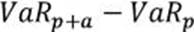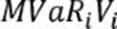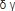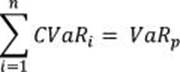## How To Pass Operational Risk Manager (ORM) 8010 Exam?

How To Pass Operational Risk Manager (ORM) 8010 Exam? The PRMIA Operational Risk Management (ORM) Certificate is designed to deliver a deep, practical understanding of operational risk management frameworks and measurement methodologies in financial institutions. FreeTestShare assists you in improving your exam preparation by providing you with the most accurate 8010 dump questions.  Our 8010 dumps are up to date and feature actual test questions and answers, allowing you to pass your exam quickly. If you prepare using 8010 test preparation material, you will pass on the first try.

Take this free 8010 practice exam right now to see how well you know yourself.

Page 1 of 6

1. When considering a request for a loan from a retail customer, which of the following factors is relevant for a bank to consider:

2. For creditrisk calculations, correlation between the asset values of two issuers is often proxied with:

3. Which of the following is not a risk faced by a bank from holding a portfolio of residential mortgages?

4. Which of the following statements are correct in relation to the financial system just prior to the current financial crisis:

I. The system was robust against small random shocks, but not against large scale disturbances to key hubs in the network

II. Financial innovation helped reduce the complexity of the financial network

III. Knightian uncertainty refers to risk that can be quantified and measured

IV. Feedback effects under stress accentuated liquidity problems

5. Which of the following statements is true:

I. When averaging quantiles of two Pareto distributions, the quantiles of the averaged models are equal to the geometric average of the quantiles of the original models based upon the number of data items in each original model.

II. When modeling severity distributions, we can only use distributions which have fewer parameters than the number of datapoints we are modeling from.

III. If an internal loss data based model covers the same risks as a scenario based model, they can can be combined using the weighted average of their parameters.

IV If an internal loss model and a scenario based model address different risks, the models can be combined by taking their sums.

6. Which of the formulae below describes incremental VaR where a new position 'm' is added to the portfolio? (where p is theportfolio, and V_i is the value of the i-th asset in the portfolio. All other notation and symbols have their usual meaning.)

A)B)C)D)7. If the odds of default are 1:5, what is the probability of default?

8. Which of the following are valid approaches to leveraging external loss data for modeling operational risks:

I. Both internal and external losses can be fitted with distributions, and a weighted average approach using these distributions is relied upon for capital calculations.

II. External loss data is used to inform scenario modeling.

III. External loss data is combined with internal loss data points, and distributions fitted to the combined data set.

IV. External loss data is used to replace internal loss data points to create a higher quality data set to fit distributions.

9. The standalone economic capital estimates for the three business units of a bank are \$100, \$200 and \$150 respectively .

What is the combined economic capital for the bank, assuming the risks of the three business units are perfectly correlated?

10. Company A issues bonds with a face value of \$100m, sold at issuance at \$98. Bank B holds \$10m in face of these bonds acquired at a price of \$70 .

What is Bank B's exposure to the debt issued by Company A?